# What are Parse Trees (Derivation Trees)?

Derivations mean replacing a given string’s non-terminal by the right-hand side of the production rule. The sequence of applications of rules that makes the completed string of terminals from the starting symbol is known as derivation. The parse tree is the pictorial representation of derivations. Therefore, it is also known as derivation trees. The derivation tree is independent of the other in which productions are used.

A parse tree is an ordered tree in which nodes are labeled with the left side of the productions and in which the children of a node define its equivalent right parse tree also known as syntax tree, generation tree, or production tree.

A Parse Tree for a CFG G =(V,∑, P,S) is a tree satisfying the following conditions −

• Root has the label S, where S is the start symbol.

• Each vertex of the parse tree has a label which can be a variable (V), terminal (Σ), or ε.

• If A → C1,C2…….Cn is a production, then C1,C2…….Cn are children of node labeled A.

• Leaf Nodes are terminal (Σ), and Interior nodes are variable (V).

• The label of an internal vertex is always a variable.

• If a vertex A has k children with labels A1,A2…….Ak,then A →

A1,A2…….Ak will be production in context-free grammar G.

Yield − Yield of Derivation Tree is the concatenation of labels of the leaves in left to right ordering.

Example1 − If CFG has productions.

S → a A S | a
S → Sb A | SS | ba

Show that S ⇒ *aa bb aa & construct parse tree whose yield is aa bb aa.

Solution

S ⇒lm lm a $\underline{A}$ S

⇒ a $\underline{S\:b}$ A S

⇒ aa b $\underline{A}$ S

⇒ aa bba $\underline{S}$

∴ S ⇒ * aa bb aa

## Derivation Tree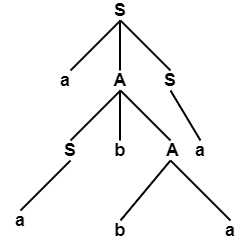Yield = Left to Right Ordering of Leaves = aa bb aa

Example2

Consider the CFG

S → bB | aA
A → b | bS | aAA
B → a |aS | bBB

Find (a) Leftmost

• Rightmost Derivation for string b aa baba. Also, find derivation Trees.

Solution

• Leftmost Derivation

S⇒b $\underline{B}$

⇒ bb $\underline{B}$B

⇒ bba$\underline{B}$

⇒ bbaa$\underline{S}$

⇒ bb aa$\underline{bB}$

⇒ bb aa b $\underline{aS}$

⇒ bb aa bab $\underline{B}$

⇒ bb aa ba ba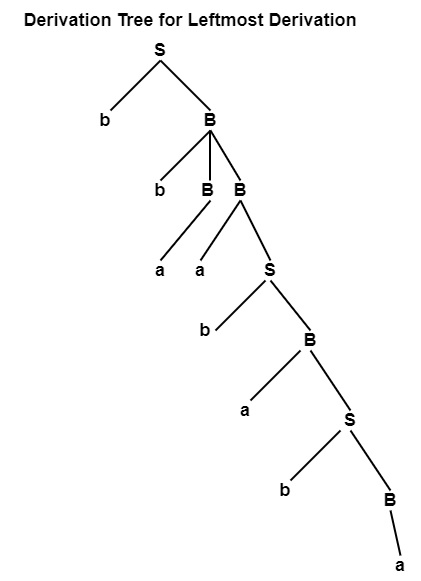• Rightmost Derivation

S ⇒ b$\underline{B}$

⇒ bb B$\underline{B}$

⇒ bbBa$\underline{S}$

⇒ bbBab$\underline{B}$

⇒ bbBaba$\underline{S}$

⇒ bbBabab$\underline{B}$

⇒ bb$\underline{B}$abab a

⇒ bbaababa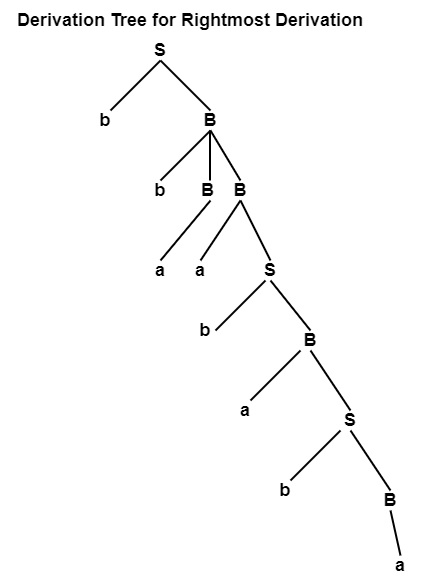Example3 − Consider the Grammar given below −

E⇒ E+E|E $\ast$ E|id

Find

• Leftmost
• Rightmost Derivation for the string.

Solutio

• Leftmost Derivation

E ⇒ $\underline{E}$+E

⇒ $\underline{E}$+E+E

⇒ id+E+E

⇒ id+id+E

⇒ id+id+id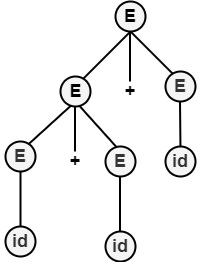• Rightmost Derivation

E ⇒ E+$\underline{E}$

⇒ E+E+$\underline{E}$

⇒ E+E+id

⇒ E+id+id

⇒ id+id+id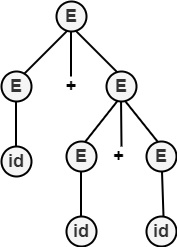Updated on: 26-Oct-2021

7K+ Views